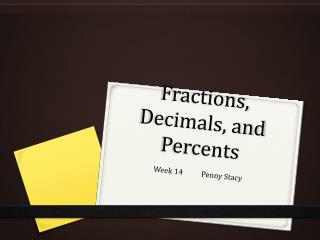DownloadDownload PresentationFractions, Decimals, and Percents

# Fractions, Decimals, and Percents

Télécharger la présentation## Fractions, Decimals, and Percents

- - - - - - - - - - - - - - - - - - - - - - - - - - - E N D - - - - - - - - - - - - - - - - - - - - - - - - - - -
##### Presentation Transcript

1. Fractions, Decimals, and Percents Week 14 Penny Stacy

2. Percents • Means per 100. • You can express a percent as a fraction with a denominator of 100. To write a percent, use the percent sign (%). • Ex. 29/100 can be written as 29%

3. Decimal to Percent • To convert a decimal to a percent, multiply the decimal by 100 and insert the percent sign. • Multiplying a decimal by 100 is like moving the decimal point 2 places to the right. Ex. What percent is equivalent to 0.37? Multiply the decimal by 100 and insert the percent sign. ( Move the decimal 2 places to the right.) 0.37 X 100 = 37 % Insert the percent sign.

4. Fraction to Decimal • 2 ways to convert a fraction to a decimal • If the denominator is a factor of a power of 10, write an equivalent fraction with a power of 10 as the denominator. Ex. ½ = ½ X 5/5 = 5/10 = 0.5 • You can also divide the numerator by the denominator. What decimal is equivalent to 3/8? 3/8 = 0.375

5. Fraction to Percent • First convert the fraction to a decimal. Then convert the decimal to a percent. • The girls’ soccer team has 16 players. Fourteen of those players are in the sixth grade. What percent of the players are in the sixth grade? Write the amount as a fraction. Then convert the fraction to a decimal; then to a percent.

6. Decimal to Fraction (in simplest form) • Write the digits of the decimal as the numerator and the least place of the decimal as the denominator. • Write the fraction in simplest form. Ex. 0.92=92/100 ÷ 4/4 =23/25 0.92 = 23/25

7. The decimals, fractions, and percents shown in this lesson are all examples of rational numbers. You can represent rational numbers on a number line. Percents greater than 100% can also be represented as decimals, whole numbers, or mixed numbers. • Ex. 1 ½ = 1.5 = 150%. • Draw number line and express the number as a fraction, decimal, and percent.

8. Apply • Ashley recorded that it rained 9 days during a 40-day period. Represent the part of the 40-day period that it rained as a decimal and as a percent. • What fraction of the days did it rain?___________ • Find the decimal first. Divide the numerator by the denominator. _____________ ÷ ________ =______ • Now find the percent.__________ • As a decimal it rained ________ of the days. • As a percent, it rained _________ of the days.